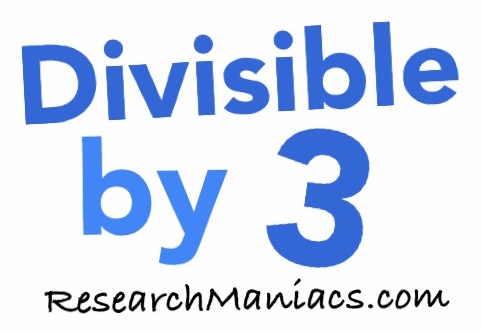Is 10 divisible by 3?Is 10 divisible by 3? In other words, if you divide 10 by 3, will you get a whole number with no remainder?

Of course, you could use a calculator to find out if 10 is divisible by 3, but what fun would that be?

To find out if 10 is divisible by 3, we will add up the numbers that make 10 as follows: 1 + 0 = 1

We know that if the sum of the numbers that make up 10 is divisible by 3, then 10 is divisible by 3.

Since the sum of the digits in 10 is not divisible by 3, 10 is also NOT divisible by 3. Thus, the answer to the question "Is 10 divisible by 3?" is as follows:

No

Note: If you divide 10 by 3, you get 3.33 which is not a whole number.

Divisible by 3?
Do you need to check another number? Enter a number below to see if it is divisible by 3.

Is divisible by 3?

Is 11 divisible by 3?
Can you do it on your own now? If not, go here for the next number on our list.